# Completed 11 out of 20 If a buffer solution is 0.170 M in a weak base...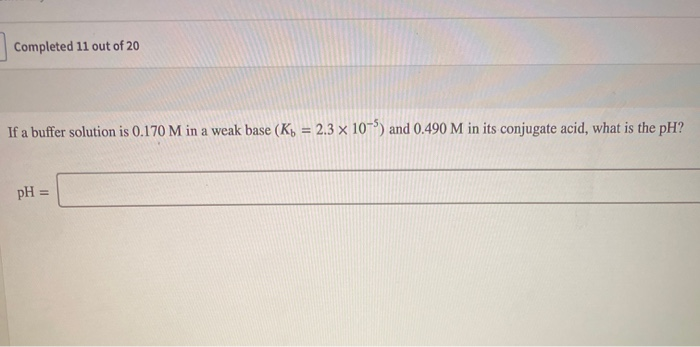Completed 11 out of 20 If a buffer solution is 0.170 M in a weak base (K) = 2.3 x 10-) and 0.490 M in its conjugate acid, what is the pH? pH =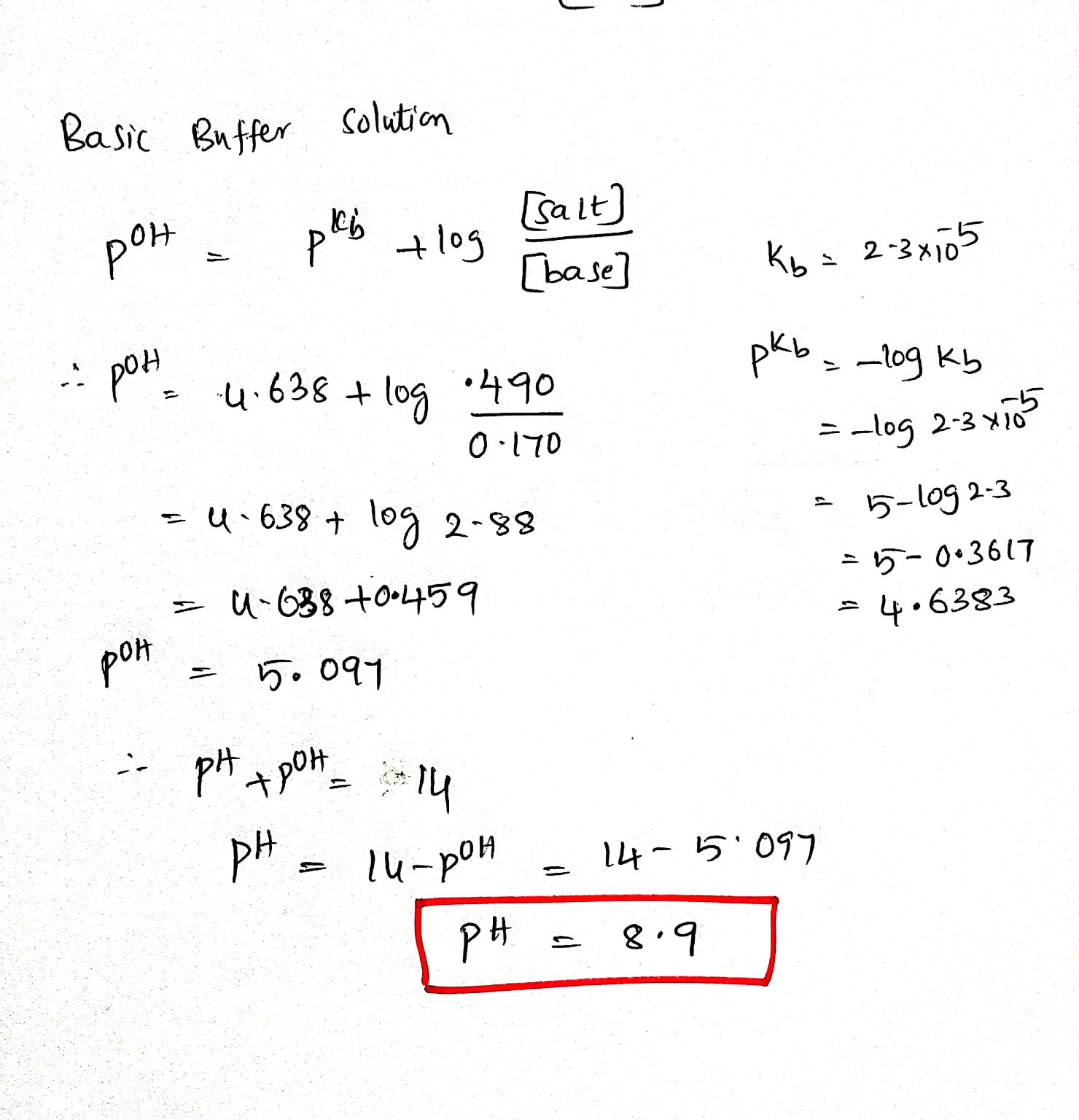#### Earn Coin

Coins can be redeemed for fabulous gifts.

Similar Homework Help Questions
• ### If a buffer solution is 0.170 M in a weak base (Kb = 8.5×10−5 M) and...

If a buffer solution is 0.170 M in a weak base (Kb = 8.5×10−5 M) and 0.480 M in its conjugate acid, what is the pH?

• ### If a buffer solution is 0.170 M in a weak acid (Ka = 7.2 x 10-5)...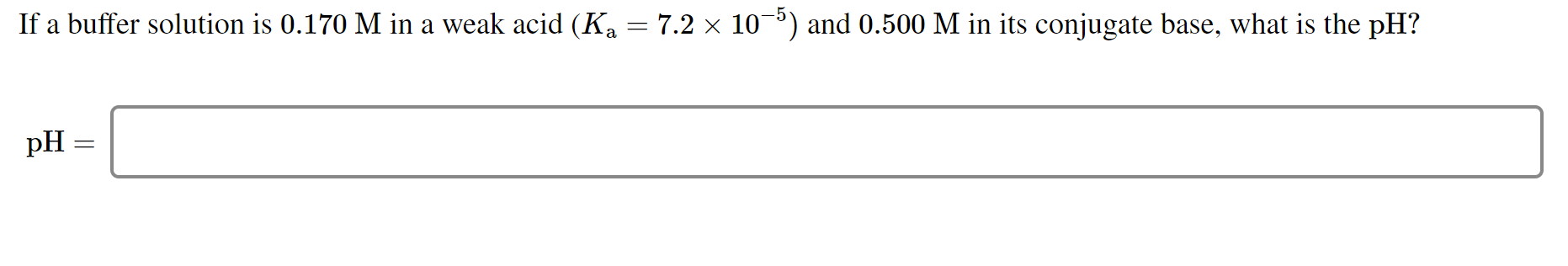If a buffer solution is 0.170 M in a weak acid (Ka = 7.2 x 10-5) and 0.500 M in its conjugate base, what is the pH? pH =

• ### If a buffer solution is 0.100 M in a weak base (Kb = 5.0 * 10%)...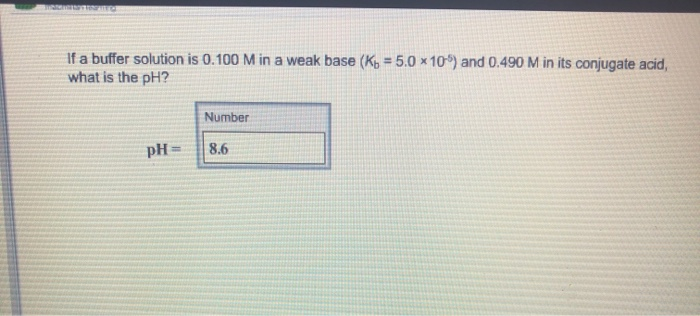If a buffer solution is 0.100 M in a weak base (Kb = 5.0 * 10%) and 0.490 M in its conjugate acid, what is the pH? Number pH = 18.6

• ### 1. If a buffer solution is 0.150 M in a weak base (K,.710 what is the...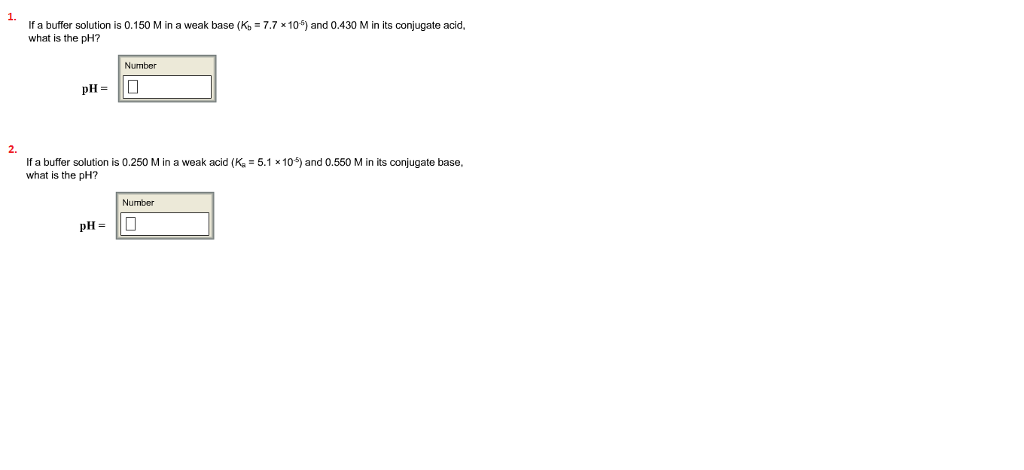1. If a buffer solution is 0.150 M in a weak base (K,.710 what is the pH? and 0.430 M in its conjugate acid Number 2. If a buffer solution is 0.250 M in a weak acid (K what is the pH? 5.1 x10) and 0.550 M in its conjugate base, Number pH-

• ### If a buffer solution is 0.220 M in a weak base (K) = 7.8 x 10...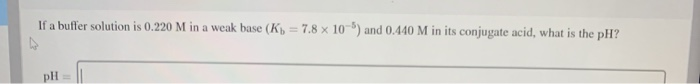If a buffer solution is 0.220 M in a weak base (K) = 7.8 x 10 ) and 0.440 M in its conjugate acid, what is the pH?

• ### If a buffer solution is 0.100 M in a weak base (K= 5.7 x 10-5) and...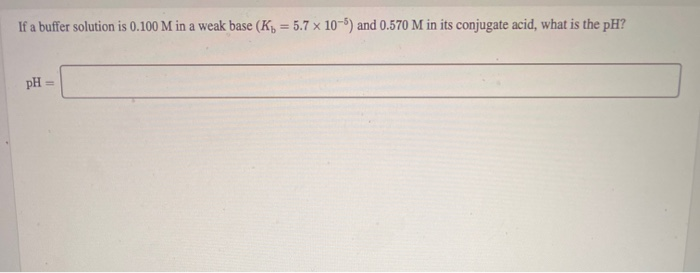If a buffer solution is 0.100 M in a weak base (K= 5.7 x 10-5) and 0.570 M in its conjugate acid, what is the pH? pH =

• ### If a buffer solution is 0.420 M in a weak acid (Ka = 5.6 × 10-6)...

If a buffer solution is 0.420 M in a weak acid (Ka = 5.6 × 10-6) and 0.170 M in its conjugate base, what is the pH?

• ### pH and buffer solutions If a buffer solution is 0.150 M in a weak acid (K,...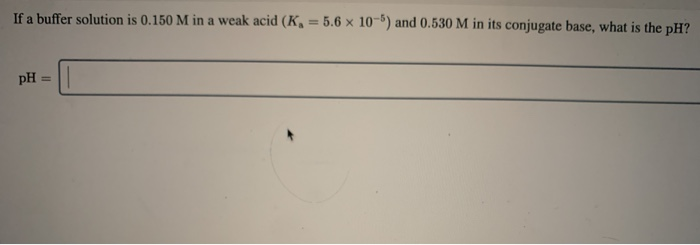pH and buffer solutions If a buffer solution is 0.150 M in a weak acid (K, = 5.6 x 10-) and 0.530 M in its conjugate base, what is the pH?

• ### pH and buffer solutions If a buffer solution is 0.570 M in a weak acid (K...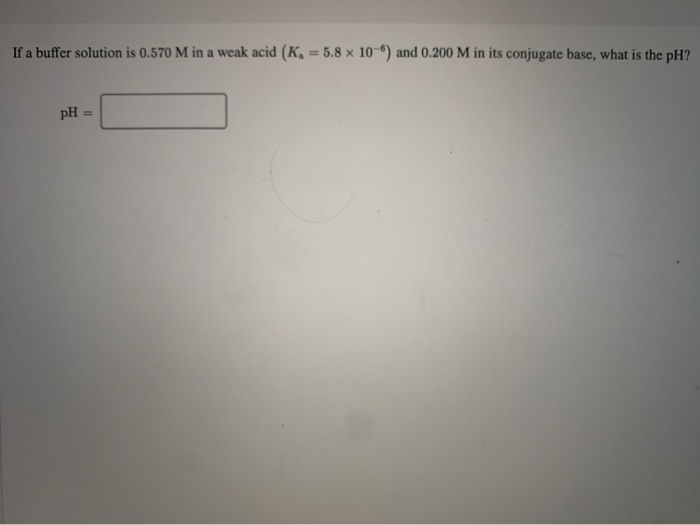pH and buffer solutions If a buffer solution is 0.570 M in a weak acid (K = 5.8 x 10"") and 0.200 M in its conjugate base. what is the H2 pH =

• ### If a buffer solution is 0.100 M in a weak base (Kb = 6.5 x 10-5)...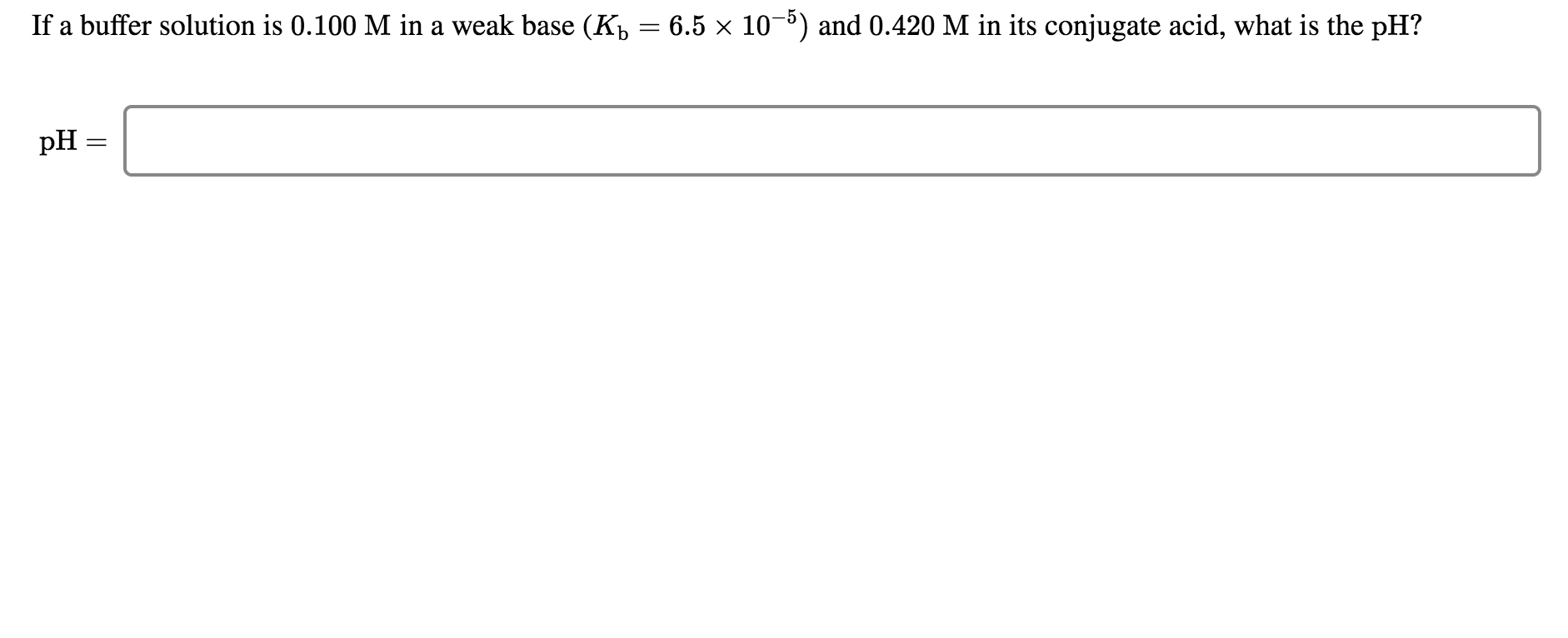If a buffer solution is 0.100 M in a weak base (Kb = 6.5 x 10-5) and 0.420 M in its conjugate acid, what is the pH? pH =